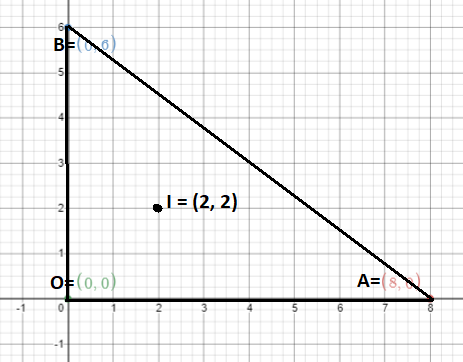Courses
Courses for Kids
Free study material
Free LIVE classes
MoreLIVE
Join Vedantu’s FREE Mastercalss

# If the line 3x + 4y – 24 = 0 intersects the x-axis at the point A and the y-axis at the point B, then the incentre of the triangle OAB, where O is the origin, is:A. (3, 4)B. (2, 2)C. (4, 4)D. (4, 3)Verified
360.6k+ views
Hint: Let us find the point where the given line intersects x-axis and y-axis (i.e. point A and point B) and then find distance between these points.

As we know that if any line intersects the x-axis at any point A then the y-coordinate of the point A will be equal to zero.
So, putting y = 0 in the given equation. We get,
3x + 0 – 24 =0
x = 8
So, coordinates of point A will be A (8, 0).
And if any line intersects the y-axis at any point B then the x-coordinate of the point B will be equal to zero.
So, putting x = 0 in the given equation. We get,
0 + 4y – 24 =0
y = 6
So, coordinates of point B will be B (0, 6).
And O is the origin. So, coordinates of O will be O (0, 0).
As we know from the properties of the triangle that incentre of any triangle XYZ having coordinates X $\left( {{{\text{x}}_{\text{1}}}{\text{, }}{{\text{y}}_{\text{1}}}} \right)$, Y $\left( {{{\text{x}}_{\text{2}}}{\text{, }}{{\text{y}}_{\text{2}}}} \right)$ and Z $\left( {{{\text{x}}_{\text{3}}}{\text{, }}{{\text{y}}_{\text{3}}}} \right)$ and length of YZ is a, length of ZX is b and the length of XY is c is given as I$\left( {{\text{h, k}}} \right)$. Where h = $\dfrac{{{\text{a}}{{\text{x}}_{\text{1}}}{\text{ + b}}{{\text{x}}_{\text{2}}}{\text{ + c}}{{\text{x}}_{\text{3}}}}}{{{\text{a + b + c}}}}$ and k = $\dfrac{{{\text{a}}{{\text{y}}_{\text{1}}}{\text{ + b}}{{\text{y}}_{\text{2}}}{\text{ + c}}{{\text{y}}_{\text{3}}}}}{{{\text{a + b + c}}}}$.
So, to find the incentre of the given triangle OAB. We had to find the length of OA, AB and BO.
And to find the distance between two points we had to use distance formula which states that if P $\left( {{{\text{x}}_{\text{1}}}{\text{, }}{{\text{y}}_{\text{1}}}} \right)$ and Q $\left( {{{\text{x}}_{\text{2}}}{\text{, }}{{\text{y}}_{\text{2}}}} \right)$ are the two given points then PQ = $\sqrt {{{\left( {{{\text{x}}_{\text{1}}}{\text{ - }}{{\text{x}}_{\text{2}}}} \right)}^{\text{2}}}{\text{ + }}{{\left( {{{\text{y}}_{\text{1}}}{\text{ - }}{{\text{y}}_{\text{2}}}} \right)}^{\text{2}}}}$
Let the length of AB be a.
So, AB = a = $\sqrt {{{\left( {{\text{8 - 0}}} \right)}^{\text{2}}}{\text{ + }}{{\left( {{\text{0 - 6}}} \right)}^{\text{2}}}} {\text{ = }}\sqrt {{\text{100}}} {\text{ = 10}}$
Let the length of OB be a.
So, OB = b = $\sqrt {{{\left( {{\text{0 - 0}}} \right)}^{\text{2}}}{\text{ + }}{{\left( {{\text{0 - 6}}} \right)}^{\text{2}}}} {\text{ = }}\sqrt {36} {\text{ = 6}}$
Let the length of AO will be c.
So, AO = c = $\sqrt {{{\left( {{\text{8 - 0}}} \right)}^{\text{2}}}{\text{ + }}{{\left( {{\text{0 - 0}}} \right)}^{\text{2}}}} {\text{ = }}\sqrt {64} {\text{ = 8}}$
Now applying Incentre formula in triangle OAB . Where ${{\text{x}}_{\text{1}}}{\text{, }}{{\text{x}}_{\text{2}}}$ and ${{\text{x}}_{\text{3}}}$ are the x-coordinates,${{\text{y}}_{\text{1}}}{\text{, }}{{\text{y}}_{\text{2}}}$ and ${{\text{y}}_{\text{3}}}$ are the y-coordinates of O, A and B.
And a, b, c is specified above.
So, Incentre = $\left( {\dfrac{{{\text{0 + 6*8 + 0}}}}{{{\text{10 + 6 + 8}}}}{\text{, }}\dfrac{{{\text{0 + 0 + 8*6}}}}{{{\text{10 + 6 + 8}}}}} \right){\text{ = }}\left( {{\text{2, 2}}} \right)$.
So, the coordinates of the incentre of triangle OAB will be (2, 2).Hence, the correct option will be B.

Note: Whenever we come up with type of problem where we are asked to find the incentre of triangle then first, we had to find the coordinates of the vertices of the triangle and then we had to find the length of its all sides after that we can apply direct formula for finding the coordinates of incentre of the triangle that is I = $\left( {\dfrac{{{\text{a}}{{\text{x}}_{\text{1}}}{\text{ + b}}{{\text{x}}_{\text{2}}}{\text{ + c}}{{\text{x}}_{\text{3}}}}}{{{\text{a + b + c}}}},{\text{ }}\dfrac{{{\text{a}}{{\text{y}}_{\text{1}}}{\text{ + b}}{{\text{y}}_{\text{2}}}{\text{ + c}}{{\text{y}}_{\text{3}}}}}{{{\text{a + b + c}}}}} \right)$.
Last updated date: 24th Sep 2023
Total views: 360.6k
Views today: 4.60k